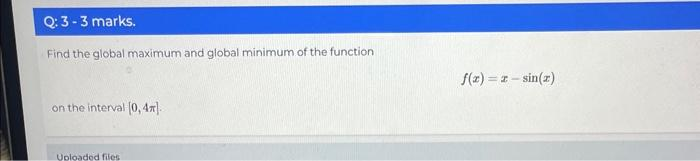# Question Find the global maximum and global minimum of the function $f(x)=x-\sin (x)$ on the interval $$[0,4 \pi]$$.Transcribed Image Text: Find the global maximum and global minimum of the function $f(x)=x-\sin (x)$ on the interval $$[0,4 \pi]$$.
Transcribed Image Text: Find the global maximum and global minimum of the function $f(x)=x-\sin (x)$ on the interval $$[0,4 \pi]$$.&#12304;General guidance&#12305;The answer provided below has been developed in a clear step by step manner.Step1/2Given the function $$\mathrm{{f{{\left({x}\right)}}}={x}-{\sin{{x}}}}$$Differentiating $$\mathrm{{f}'{\left({x}\right)}={1}-{\cos{{x}}}}$$Explanation:Please refer to solution in this step.Step2/2For critical points \( \mathrm{{f}'{\left({x}\right)} ... See the full answer